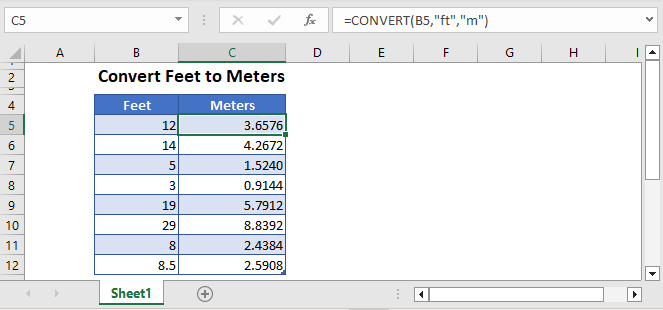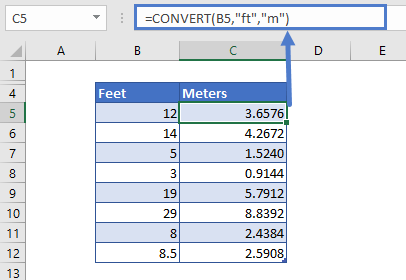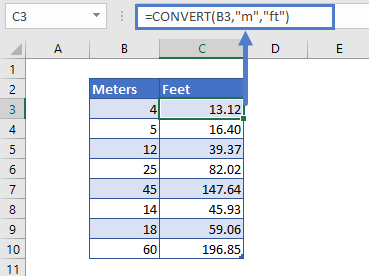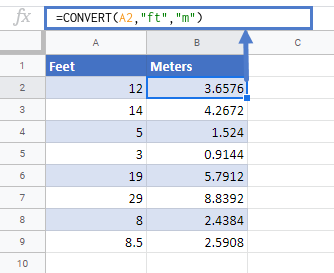# Convert Feet to Meters in Excel & Google Sheets

This tutorial will demonstrate how to convert values given in feet to meters in Excel & Google Sheets.## Using the CONVERT Function

The CONVERT function can be used to convert values given in feet to meters. We just need to enter both the value and measurement types into the function. We can use the abbreviations of the measurements.

Let’s test the formula on an example for better understanding. Say in column B we have some values given in feet that need to be converted to meters. So, just type the following formula in cell C5 and drag it down:

``=CONVERT(B5,"ft","m")``By changing the values in Column B, the Column C values will update automatically.

## Conversion of Values in meters to feet

Just by switching the measurements arguments, we can convert the values given in meters to feet:

``=CONVERT(B3,"m","ft")``## Convert Values in Feet to Meters in Google Sheets

The conversion of feet to meters using the CONVERT Function works the same in Google Sheets as in Excel:### Excel Practice Worksheet

Practice Excel functions and formulas with our 100% free practice worksheets!